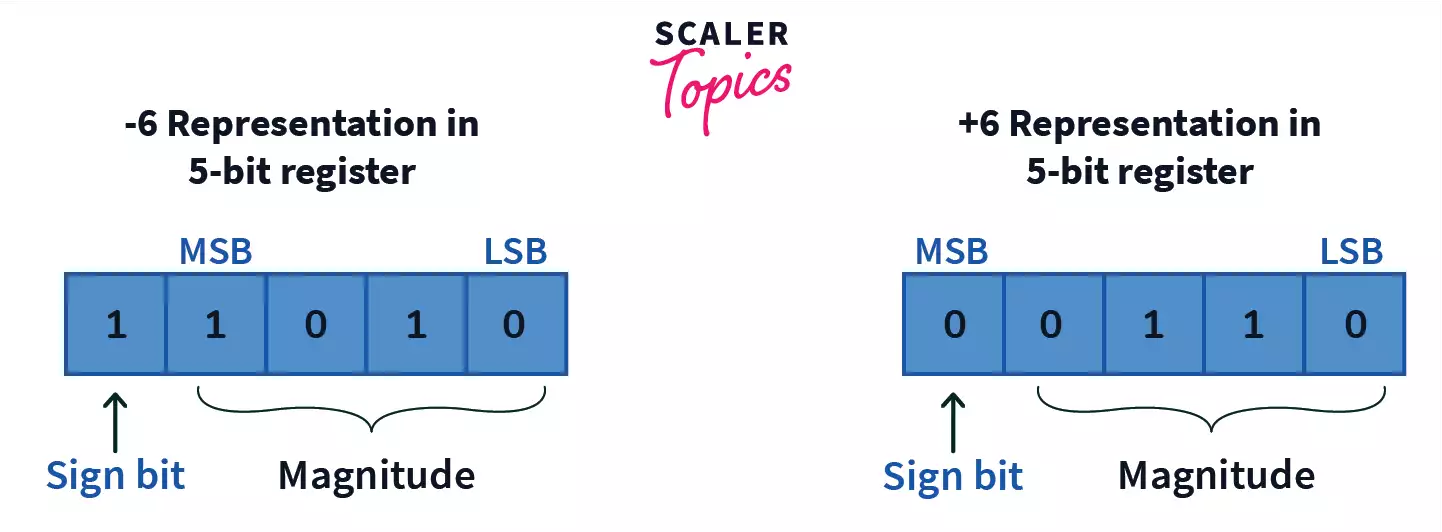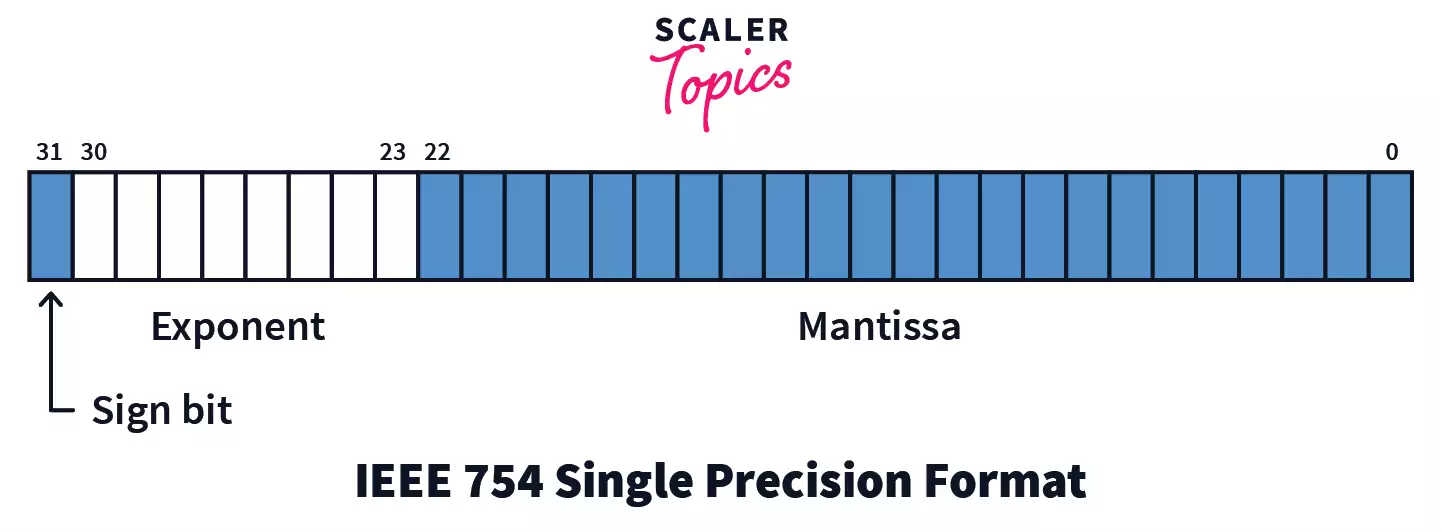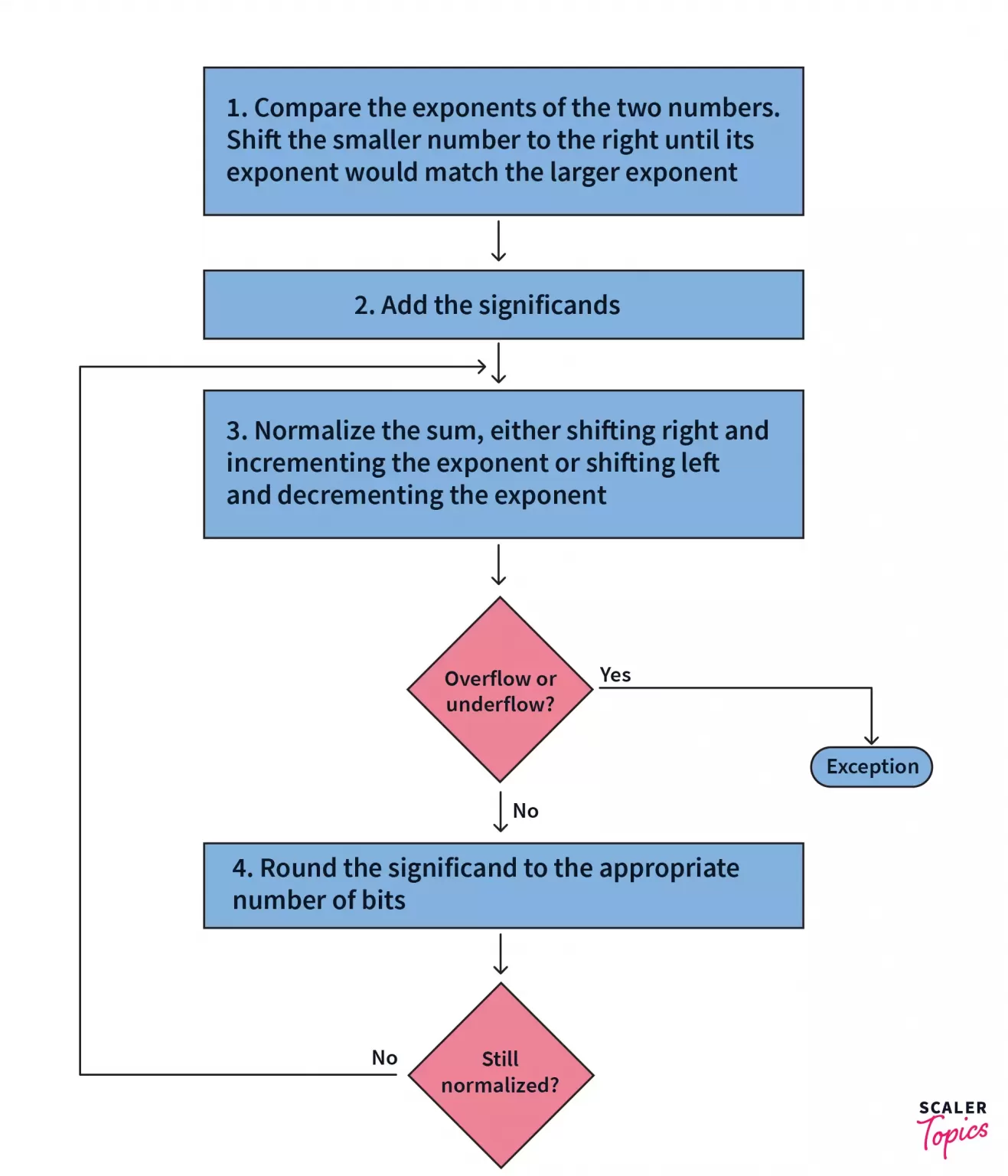# Binary Subtraction

Learn binary subtraction of two numbers using 1's and 2's complement.

Before we begin, let’s look at the following operation:

KaTeX parse error: Expected 'EOF', got ' ' at position 1:  ̲ 55 – 12 = 43

In the above operation, a number 12 is being subtracted from another number 55 to give a difference of 43.

Manually, it can be done easily using arithmetic operations in the decimal system (base-10), a high level language. But computers operate using machine language or machine code which is a low level language that is composed of the binary (base-2) digits 0 and 1.

Processing is done in computers in binary. Binary is a number system of base-2 notation that uses only 2 states: 0 and 1. A decimal number can be uniquely constructed in binary using a combination of 0’s and 1’s.

The numbers in the above operation can be denoted in binary as follows:

KaTeX parse error: Expected 'EOF', got '\\' at position 27: …> (110111)_{2} \̲\̲ (12)_{10} -> (…

On subtraction, the difference $(43)_{10}$ would be represented as:

KaTeX parse error: Expected 'EOF', got '–' at position 14: (110111)_{2} –̲ (001100)_{2} =…

But how does this subtraction take place inside a computer system? We will be exploring it in this article.

## Taking 1’s And 2’s Complement

Before we start binary subtraction, we first need to understand what 1’s and 2’s complement is.

All information in the system memory is stored in binary, meaning all integers are stored in the memory in various combinations of 0’s and 1’s.

While it is pretty easy to store positive numbers, negative numbers is where it gets a little tricky. Storing negative numbers as 0’s and 1’s, and ensuring they aren’t taken as their positive counterparts, requires us to tweak the system that dictates how numbers are represented in binary.

1’s complement is a system where negative numbers are represented with the inverse of their binary representation of the corresponding positive numbers. It enables negative numbers to be denoted in binary form.

What we are doing here, is essentially “flipping” the roles of 0 and 1. Hence instead of starting with 0000 as we normally do, we start from 1111.

Therefore, negative numbers can be represented like this: $(1111)_{2}$ is $(-1)_{10}$ $(1110)_{2}$ is $(-2)_{10}$ $(1101)_{2}$ is $(-3)_{10}$

And so on all the way to $(1000)_{2}$ which represents $(-8)_{10}$

1’s complement is taken by inverting all the binary digits in the number, i.e. replacing 0’s with 1’s and 1’s with 0’s.

For example, the 1’s complement of $(100101)_{2}$ is $(011010)_{2}$

2’s complement on the other hand enables us to denote both positive numbers and negative numbers in the binary system. The most significant bit in the 2’s complement representation of a binary number denotes its sign, i.e. whether it is positive or negative.

2’s complement of a number can be found by adding a 1 to the 1’s complement of a number.

From the example above, we find the 1’s complement of $(100101)_{2}$ to be $(011010)_{2}$

Now, the 2’s complement of $(100101)_{2}$ is $(011011)_{2}$

A point to note is that 1’s and 2’s complement are used to represent signed numbers. Hence this means that the Most Significant Bit (MSB) of the 1’s complement or 2’s complement of a number represents the sign of the number. MSB denotes the second leftmost bit of a number denoted in binary form, after the sign bit. An MSB of 0 denotes a positive number and an MSB of 1 represents a negative number.As we can see above, the number $(-6)_{10}$ will be denoted as $(11010)_{2}$ and $(6)_{10}$ will be denoted as $(00110)_{10}$. This is because we count upwards from 0000 for positive integers and downwards from 1111 for negative integers.

## Binary Subtraction

In computers, subtraction of numbers is done using addition of one number with the 2’s complement of the other.

For example:

KaTeX parse error: Expected 'EOF', got '’' at position 15: (X-Y) = X + (2’̲s complement of $Y)$

Hence, now the problem is now simplified to the addition of two numbers.

One important thing to note is that both the binary numbers must have the same number of bits. If they do not, then we need to pad the shorter number with 0’s to its left until both numbers are equal.

In the example we’ve taken in the beginning, the binary representation of 53 is $(110101)_{2}$, which has 6 bits and the binary representation of 12 is $(1101)_{2}$, which has 4 bits. Hence an extra two 0’s were added to $(1101)_{2}$ at the beginning to make it $(001101)_{2}$.

Furthermore, an additional two 0’s were padded to both the numbers to make the number of bits in the numbers divisible by 8.

The addition of binary bits is as follows:

XYX+YX-Y
0000
0111(the 1 iscarried over)
1011
110 (the 1 is carried over)0

An interesting point to note from the table above is that binary addition and subtraction is the same as XOR operation. The computer uses this property to add and subtract binary numbers.

2’s complement changes the sign of the number. For example, the 2’s complement of 12 is taken as below:

KaTeX parse error: Expected 'EOF', got '—' at position 10: (001100) —̲> (110011) —> (…

Now if we observe closely, we can see that these operations resemble XOR operations. In fact, binary subtraction is just repeated XOR operation while keeping track of the carryovers.

## An Example

As an example, let us subtract 5 from 7.

i) Binary representation of 5 is $(0101)_{2}$. The 1’s complement of 5 is then: $(1010)_{2}$

ii) 2’s complement of 5 = (1’s complement of 5 + 1) = $(1010 + 1)_{2}$ = $(1011)_{2}$.

iii) Now, 7 + (-5) = 0111 + 1011 = (1)0010

As we’ve said before, we will be ignoring the overflow bit (1 in this case). We take the rest of the bits $(0010)_{2}$, whose decimal notation is 2, which is our answer.

## Implementations

The C code to find the difference of two given numbers in different cases is as follows:

i) Code to subtract two integers:

#include <stdio.h>
#include <stdlib.h>
int main()
{
int firstNumber, secondNumber;
int sub;

printf("\nEnter the first number: ");
scanf("%d", &firstNumber);

printf("\nEnter the second number: ");
scanf("%d", &secondNumber);

sub = binSub(firstNumber, secondNumber);

printf("\nThe difference between first number and second number is: %d\n", sub);
return 0;
}
int binSub(int a, int b)
{
int carry;

while(b != 0)
{
carry = (a & b) << 1;
a = a ^ b;
b = carry;
}
return a;

}
{
int carry;
while(y != 0)
{
carry = (x & y) << 1;
x = x ^ y;
y = carry;
}
return x;
}



## Binary Subtraction of Floating Point numbers

While subtracting two integer numbers is easy as shown above, subtraction of floating point numbers is where it gets complicated.

The IEEE 754 single precision format is a scientific notation that deals with the representation of floating point numbers in binary format.

The IEEE-754 format looks like this:A. The sign bit denotes the sign of the number. A ‘0’ implies the number is positive, and a ‘1’ implies the number is negative.

B. The next 8 bits represent the exponent of the number. A point to note is that ‘127 is a unique number in 32 bit floating point binary representation’. This is known as the ‘bias’, which is calculated with the formula: $2^{k-1}-1$, where ‘k’ stands for the number of bits in the exponent field.

Hence, the bias for 32 bit floating point binary numbers is $2^{32-1}-1$ = 127.

Let’s take an example of 01000001110100000000000000000000

The sign bit is 0 and the exponent is $(10000011)_{2}$, which denotes $(131)_{10}$.

Now removing bias, 131 – 127 = 4.

Hence, the exponent of the number is $2^{4}$ = 16.

C. The final 23 bits represent the mantissa, where the 22nd bit is always ‘1’, followed by the fractional part of the number.

Let’s take an example: 01000001110100000000000000000000

The mantissa can be calculated like this: 1*(0.5) + 0*(0.25) + 1*(0.125) + … + = 0.625

Therefore, the mantissa will be 1 + 0.625 (The ‘1’ is the 22nd bit)

= 1.625

Therefore, the decimal notation of the 32 bit floating point binary number 01000001110100000000000000000000 is: $(-1)^{sign} * (exponent) * (mantissa)$

= $(-1)^{0}$ * (16) * (1.625) = 26

As mentioned before, the sign bit at the MSB position denotes the sign of the number, with 0 representing positive and 1 representing negative. It is then followed by the exponent value of the digit and then the mantissa.

A number is said to be normalized if it has no leading zeros. Hence, 1.0 * 104 is normalized whereas 0.1 * $10^{5}$ is not.

There are two important things to make sure before subtracting two floating point binary numbers: both of them must be normalized and both of them must have the same exponent.

We shall now take an example to show how it is done.

Let us see how to subtract $(0110000010)_{2}$ from $(0111000011)_{2}$ We’ll assume both numbers are in floating point binary format with 6 bits for mantissa and 4 bits for exponent. Both the numbers are in 2’s complement.

First, we write down the subtraction statement:

KaTeX parse error: Expected 'EOF', got '–' at position 18: …110000010)_{2} –̲ (0111000011)_{…

Next, we write in exponent form. To do this, we move the decimal point from the leftmost bit to the right till we encounter a ‘1’, all the while increasing the exponent value by 1. Since we are dealing with binary numbers, the exponent will be raised to 2.

KaTeX parse error: Expected 'EOF', got '–' at position 17: ….11100 * 2^{3} –̲ 0.11000 * 2^{2…

Since the exponents aren’t equal, we increase the exponent of the subtrahend:

KaTeX parse error: Expected 'EOF', got '–' at position 17: ….11100 * 2^{3} –̲ 0.01100 * 2^{3…

Now, we negate the mantissa of the second number. We do this by inverting the bits and adding one, i.e. taking 2’s complement:

$1.10011 +1= 1.10100$

Now, we add the mantissas together:

$0.11100 * 2^{3} + 1.10100 * 2^{3} + 0.11100 + 1.10100 =(1)0.10000$

We have a carryover of 1, to which we will get back to in the moment. The result now is $0.10000 * 2^{3}$

Now removing the decimal point and converting it to binary, we get:

010000 0011